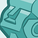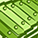# QlikView App Dev

Discussion Board for collaboration related to QlikView App Development.

Announcements
cancel
Showing results for
Did you mean:Contributor

I have the following load script which allows the user to define a folder on their computer, subsequently loading each of the workbooks and worksheets from the folder into QlikView (assuming that each worksheet has the same dimensions/columns).

The code executes correctly, however, how would I need to change it if I only want to extract the worksheets (Q1.1, Q1.2, Q3.3, Q2.4) instead of all the worksheets in each folder.

let vFolder = 'C:\Users\Desktop\Interest_Test\';
//Define the folder containing at data files

for each vFile in filelist('\$(vFolder)*.xlsm')
//Repeat the code for each file in the folder

OLEDB CONNECT32 TO [Provider=MSDASQL.1;Persist Security Info=False;Extended Properties="DSN=Excel Files;DBQ=\$(vFile);DriverId=1046;MaxBufferSize=2048;PageTimeout=5;"];
//Connect with each Excel file in the folder

Temp_Tables:
sqltables;

let vFileName = mid(vFile, index(vFile, '\', -1) + 1);
//Extract the Workbook name

for iSheet = 0 to NoOfRows('Temp_Tables') - 1
let vSheetName = peek('TABLE_NAME', iSheet, 'Temp_Tables');
let vSheetName = replace(replace(vSheetName, '\$', ''), chr(39), '');  // sqltables seems to add a random \$ sign and single quotes
//Extract each Sheet name

SampleData:
1
as SampleCount,
Year as [Year],
Region as [Region],
Product as [Product],
[Product Type] as [Product Type],
Cashflow as [Cashflow],
Scenario as [Scenario],
'\$(vFileName)'
as [File Name],
'\$(vSheetName)'
as [Sheet Name]
FROM [\$(vFile)]
(
ooxml, embedded labels, table is [\$(vSheetName)]);

next
//Move to the next Sheet in the workbook

DROP TABLE Temp_Tables;

next
//Repeat for the next Workbook

1 Solution

Accepted SolutionsChampion

Try,

let vFolder = 'C:\Users\Desktop\Interest_Test\';
//Define the folder containing at data files

for each vFile in filelist('\$(vFolder)*.xlsm')
//Repeat the code for each file in the folder

OLEDB CONNECT32 TO [Provider=MSDASQL.1;Persist Security Info=False;Extended Properties="DSN=Excel Files;DBQ=\$(vFile);DriverId=1046;MaxBufferSize=2048;PageTimeout=5;"];
//Connect with each Excel file in the folder

Temp_Tables:
sqltables;

let vFileName = mid(vFile, index(vFile, '\', -1) + 1);
//Extract the Workbook name

for iSheet = 0 to NoOfRows('Temp_Tables') - 1
let vSheetName = peek('TABLE_NAME', iSheet, 'Temp_Tables');
let vSheetName = replace(replace(vSheetName, '\$', ''), chr(39), '');  // sqltables seems to add a random \$ sign and single quotes
//Extract each Sheet name

If (WildMatch(vSheetName,'*Q1.1*','*Q1.2*','*Q3.3*','*Q2.4*')) Then

SampleData:
1
as SampleCount,
Year as [Year],
Region as [Region],
Product as [Product],
[Product Type] as [Product Type],
Cashflow as [Cashflow],
Scenario as [Scenario],
'\$(vFileName)'
as [File Name],
'\$(vSheetName)'
as [Sheet Name]
FROM [\$(vFile)]
(
ooxml, embedded labels, table is [\$(vSheetName)]);

EndIf
next
//Move to the next Sheet in the workbook

DROP TABLE Temp_Tables;

next

4 RepliesPartner

Hi Michael,

If it's the same fixed list of sheet names why don't you just use an inline load?

Sheetlist:

Sheet

Q1.1

Q1.2

Q3.3

Q2.4

];

let vFolder = 'C:\Users\Desktop\Interest_Test\';

//Define the folder containing at data files

for each vFile in filelist('\$(vFolder)*.xlsm')

//Repeat the code for each file in the folder

OLEDB CONNECT32 TO [Provider=MSDASQL.1;Persist Security Info=False;Extended Properties="DSN=Excel Files;DBQ=\$(vFile);DriverId=1046;MaxBufferSize=2048;PageTimeout=5;"];

//Connect with each Excel file in the folder

Temp_Tables:

sqltables;

let vFileName = mid(vFile, index(vFile, '\', -1) + 1);

//Extract the Workbook name

for iSheet = 0 to NoOfRows('Temp_Tables') - 1

let vSheetName = peek('Sheet', iSheet, 'Sheetlist');

////Old Methodology

//let vSheetName = peek('TABLE_NAME', iSheet, 'Temp_Tables');

//let vSheetName = replace(replace(vSheetName, '\$', ''), chr(39), '');  // sqltables seems to add a random \$ sign and single quotes

//Extract each Sheet name

SampleData:

1 as SampleCount,

Year as [Year],

Region as [Region],

Product as [Product],

[Product Type] as [Product Type],

Cashflow as [Cashflow],

Scenario as [Scenario],

'\$(vFileName)' as [File Name],

'\$(vSheetName)' as [Sheet Name]

FROM [\$(vFile)]

(ooxml, embedded labels, table is [\$(vSheetName)]);

next

//Move to the next Sheet in the workbook

DROP TABLE Temp_Tables;

next

//Repeat for the next WorkbookChampion

Try,

let vFolder = 'C:\Users\Desktop\Interest_Test\';
//Define the folder containing at data files

for each vFile in filelist('\$(vFolder)*.xlsm')
//Repeat the code for each file in the folder

OLEDB CONNECT32 TO [Provider=MSDASQL.1;Persist Security Info=False;Extended Properties="DSN=Excel Files;DBQ=\$(vFile);DriverId=1046;MaxBufferSize=2048;PageTimeout=5;"];
//Connect with each Excel file in the folder

Temp_Tables:
sqltables;

let vFileName = mid(vFile, index(vFile, '\', -1) + 1);
//Extract the Workbook name

for iSheet = 0 to NoOfRows('Temp_Tables') - 1
let vSheetName = peek('TABLE_NAME', iSheet, 'Temp_Tables');
let vSheetName = replace(replace(vSheetName, '\$', ''), chr(39), '');  // sqltables seems to add a random \$ sign and single quotes
//Extract each Sheet name

If (WildMatch(vSheetName,'*Q1.1*','*Q1.2*','*Q3.3*','*Q2.4*')) Then

SampleData:
1
as SampleCount,
Year as [Year],
Region as [Region],
Product as [Product],
[Product Type] as [Product Type],
Cashflow as [Cashflow],
Scenario as [Scenario],
'\$(vFileName)'
as [File Name],
'\$(vSheetName)'
as [Sheet Name]
FROM [\$(vFile)]
(
ooxml, embedded labels, table is [\$(vSheetName)]);

EndIf
next
//Move to the next Sheet in the workbook

DROP TABLE Temp_Tables;

nextContributor
Author

Worked perfectly!

Thank you for your help; going to make the Load script much more efficient!ChampionYou can also define the sheet names in a variable like below.

```//********************************************************
//Define the folder path and sheet names

Let vFolder = 'C:\Users\Desktop\Interest_Test\';
Set vSheets = 'Q1.1','Q1.2','Q3.3','Q2.4';
//********************************************************

for each vFile in filelist('\$(vFolder)*.xlsm')
//Repeat the code for each file in the folder

OLEDB CONNECT32 TO [Provider=MSDASQL.1;Persist Security Info=False;Extended Properties="DSN=Excel Files;DBQ=\$(vFile);DriverId=1046;MaxBufferSize=2048;PageTimeout=5;"];
//Connect with each Excel file in the folder

Temp_Tables:
sqltables;

let vFileName = mid(vFile, index(vFile, '\', -1) + 1);
//Extract the Workbook name

for iSheet = 0 to NoOfRows('Temp_Tables') - 1
let vSheetName = peek('TABLE_NAME', iSheet, 'Temp_Tables');
let vSheetName = replace(replace(vSheetName, '\$', ''), chr(39), '');  // sqltables seems to add a random \$ sign and single quotes
//Extract each Sheet name

If Match(vSheetName,\$(vSheets)) Then

SampleData:
1 as SampleCount,
Year as [Year],
Region as [Region],
Product as [Product],
[Product Type] as [Product Type],
Cashflow as [Cashflow],
Scenario as [Scenario],
'\$(vFileName)' as [File Name],
'\$(vSheetName)' as [Sheet Name]
FROM [\$(vFile)]
(ooxml, embedded labels, table is [\$(vSheetName)]);

EndIf

next
//Move to the next Sheet in the workbook

DROP TABLE Temp_Tables;

next

```